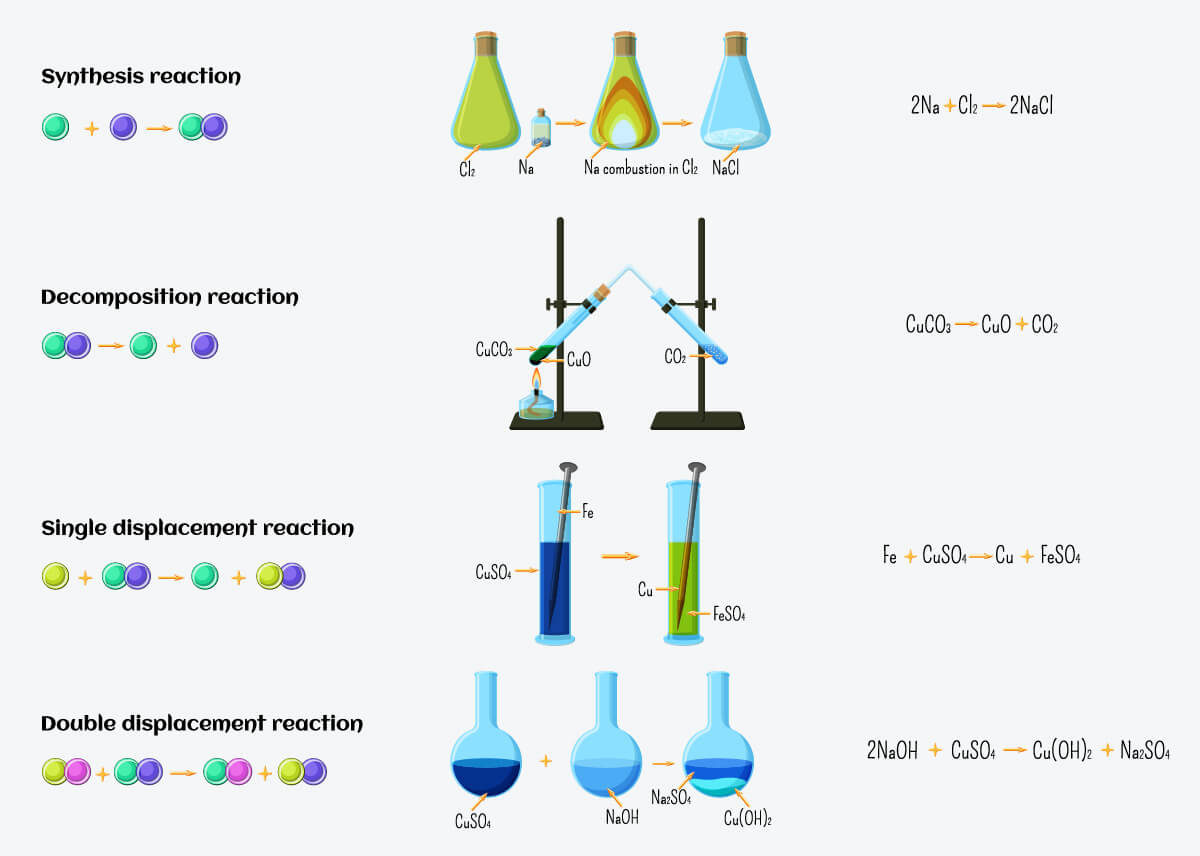Learning Objective

In this lesson we will learn how the products of chemical reactions can often be predicted, by classifying reactions into different types, based on the way atoms are rearranged.

Learning Outcomes

By the end of this lesson you will be able to:

• Identify the following types of chemical reactions:
• Combination
• Decomposition
• Single displacement
• Double displacement (precipitation and neutralisation)
• Combustion (complete and incomplete)
• Redox
• Predict the products of the following types of chemical reactions:
• Single displacement
• Precipitation
• Neutralisation
• Complete combustion.
• Identify the reducing agent and the oxidising agent in a redox reaction.

Types of Chemical Reactions

1 | Overview of Chemical Reactions

2 | Combination (Synthesis) Reactions

3 | Decomposition Reactions

4 | Displacement (Replacement) Reactions

5 | Combustion Reactions

6 | Redox Reactions

7 | Summary

Click images to preview the worksheet for this lesson and the Year 10 Chemistry Workbook (PDF and print versions)

## Overview of Chemical Reactions

• There are many different chemical reactions that exist.
• Chemical reactions can be categorised based on the way atoms and molecules are rearranged during the reaction.
• By classifying chemical reactions, it is possible to predict products, based on generalised chemical equations.
• The main types of chemical reactions are:
• Combination reactions.
• Decomposition reactions.
• Displacement reactions.
• Combustion reactions.
• Reactions can be classified on more than one way.
• Redox reactions are a particular type of reaction which may include any of the above.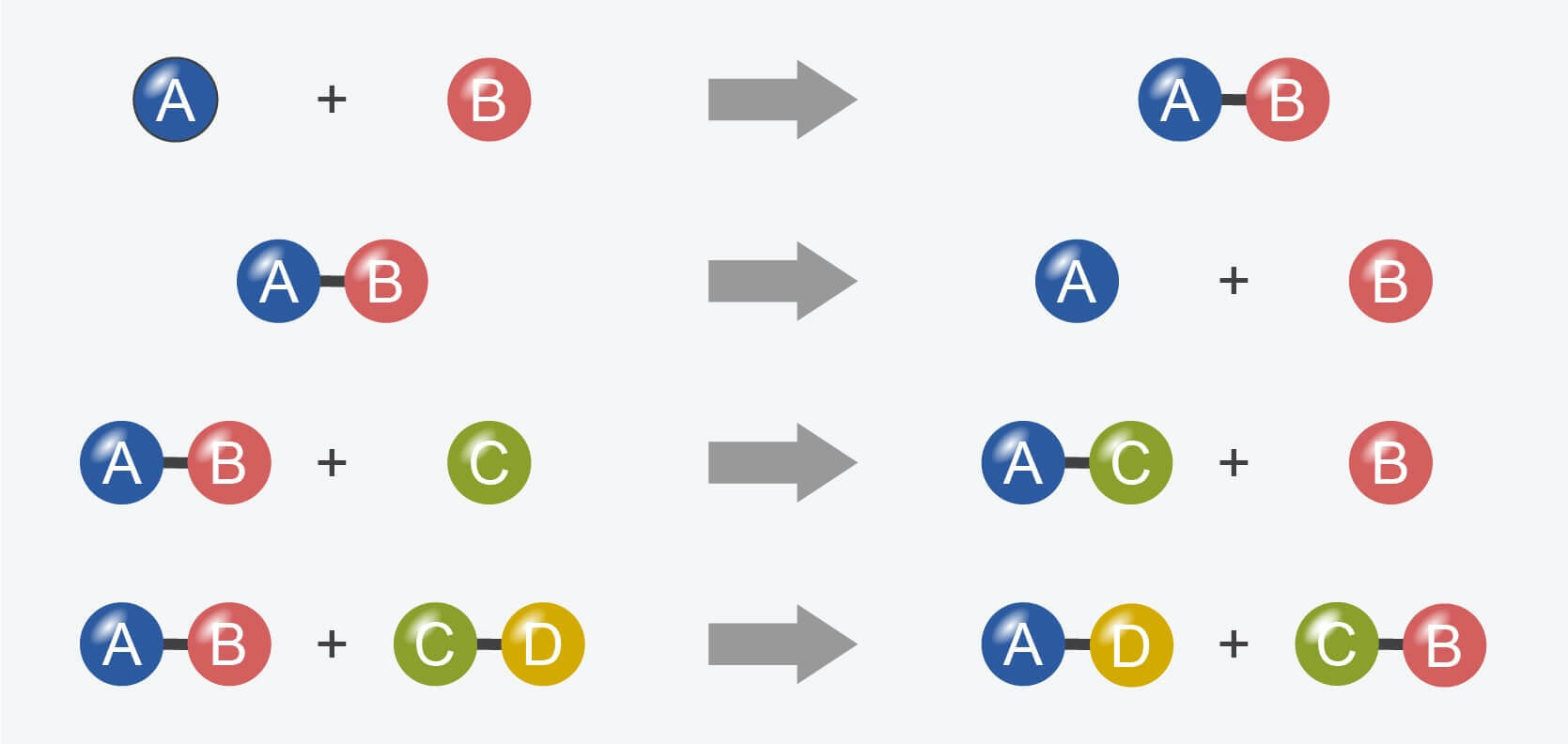Chemical reactions can be classified by the way atoms are rearranged.

(Image: Daniele Pugliesi, Wikimedia Commons)

## Combination (Synthesis) Reactions

• Combination reactions involve two or more reactants combining to form a single product.
• They can be generalised by the following equation:• Examples of combination reactions:
• Two elements combining to form a compound:
2 H2 (g)  +  O2 (g)    2 H2O (l)
• An element and a compound combining to form a new compound:
2 CO (g)  +  O2 (g)    2 CO2 (g)
• Two compounds combining to form a new compound:
Li2O (s)  +  CO2 (g)    Li2CO3 (s)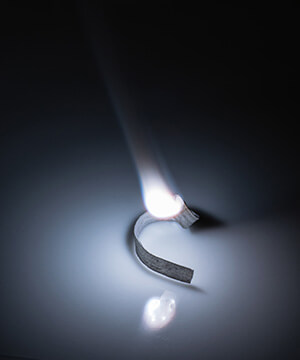When magnesium burns in air it undergoes a combination reaction with oxygen to form magnesium oxide.

## Decomposition Reactions

• Decomposition reactions involve a single reactant breaking down to form two or more products.
• They can be generalised by the following equation: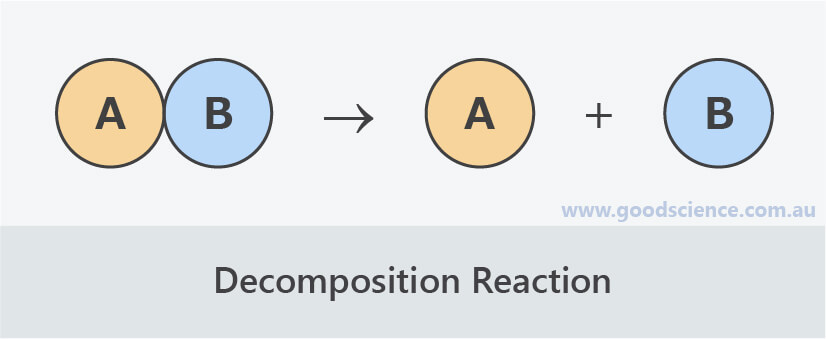• Examples of decomposition reactions:
• A compound decomposing to form two elements:
Mg3N2 (s)    3 Mg (s)  +  N2 (g)
• A compound decomposing to form a new compound and an element:
2 KClO3 (s)    2 KCl (s)  +  3 O2 (g)
• A compound decomposing to form two new compounds:
NH4NO3 (s)    N2O (g)  +  2 H2O (l)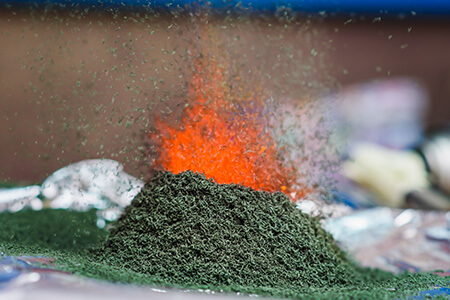When heated, ammonium dichromate decomposes into chromium (III) oxide, nitrogen gas and water vapor.

## Displacement (Replacement) Reactions

• Displacement reactions involve the displacing of one type of atom by another.
• There are two types of displacement reactions: single displacement and double displacement.

## Single Displacement (Single Replacement) Reactions

• Single displacement reactions involve an element and compound reacting to form an element and compound:
• element-1  +  compound-1    compound-2  +  element-2
• They can be generalised by the following equation: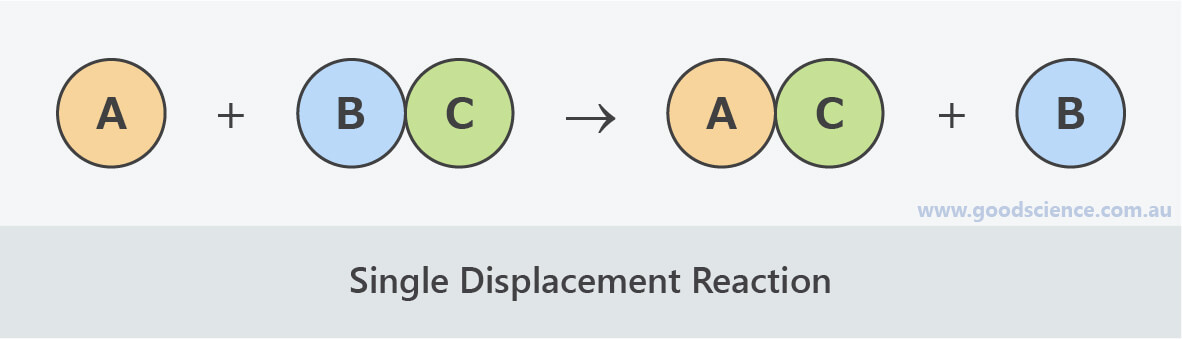• Examples of single displacement reactions:
• A metal displaces another metal in a compound:
Zn (s)  +  Cu(NO3)2 (aq)    Zn(NO3)2 (aq)  +  Cu (s)
• A metal displaces hydrogen in an acid or water:
Zn (s)  +  2 HBr (aq)    ZnBr2 (aq)  +  H2 (g)
2 Na (s)  +  2 H2O (l)    2 NaOH (aq)  +  H2 (g)
• A non-metal displaces another non-metal in a compound:
Cl2 (g)  +  2KI (aq)    2KCl (aq)  +  I2 (s)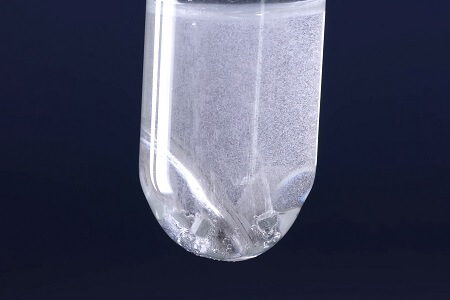Acid-metal reactions are a type of single displacement reaction.

## Double Displacement (Double Replacement) Reactions

• Double displacement reactions involve two compounds reacting to form two compounds:
• compound-1  +  compound-2    compound-3  +  compound-4
• They can be generalised by the following equation: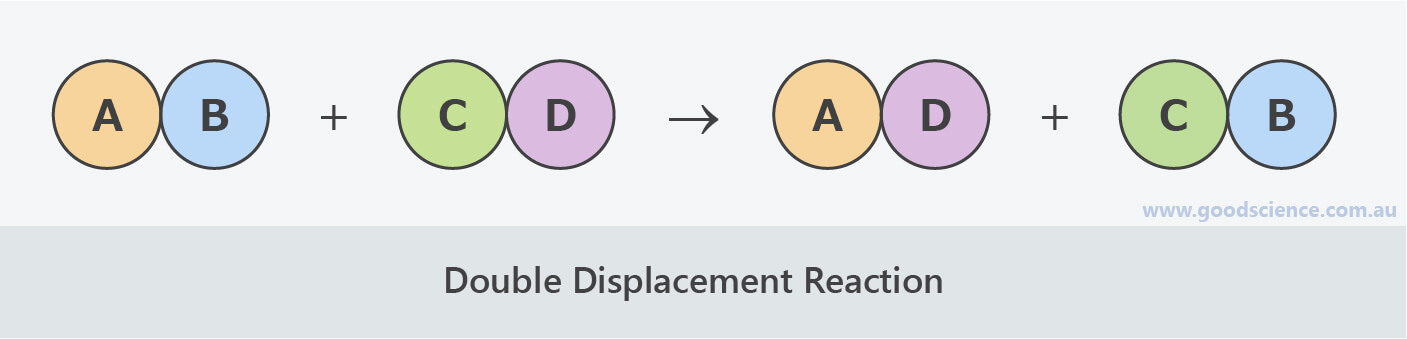• Double displacement reactions include:
• Precipitation reactionstwo soluble compounds in aqueous solution combining to form an insoluble compound and one other compound.
• Neutralisation reactions – an acid and base combining to form a salt and water.
• Examples of double displacement reactions:
• Precipitation reaction:
AgNO3 (aq)  +  NaCl (aq)    AgCl (s)  +  NaNO3 (aq)
• Neutralisation reaction:
H2SO4 (aq)  +  2 NaOH (aq)    Na2SO4 (aq)  +  2 H2O (l)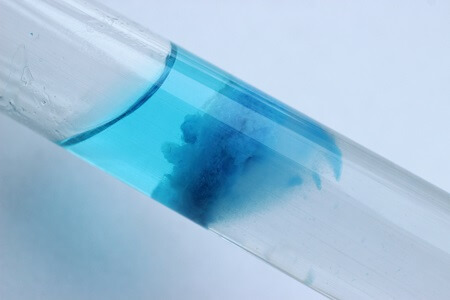Precipitation reactions are a type of double displacement reaction.

## Combustion Reactions

• Combustion reactions involve the burning of hydrocarbons (compounds containing carbon and hydrogen) with oxygen.
• The products of combustion reactions vary, depending on the supply of oxygen.

## Complete Combustion

• Complete combustion involves the burning of hydrocarbons where the supply of oxygen is not limited.
• The products of complete combustion reactions are carbon dioxide and water.
• They can be generalised by the following equation: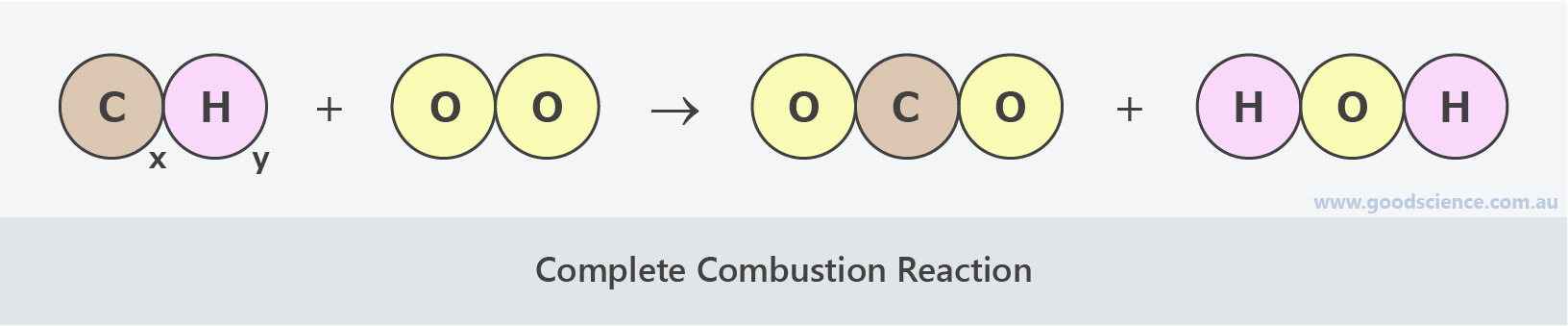• Examples of complete combustion reactions:
• Complete combustion of methane:
CH4 (g)  +  2 O2 (g)    CO2 (g)  +  2 H2O (g)
• Complete combustion of butane:
2 C4H10 (g)  +  13 O2 (g)    8 CO2 (g)  +  10 H2O (g)
• Complete combustion of ethylene:
C2H4 (g)  +  3 O2 (g)    2 CO2 (g)  +  2 H2O (g)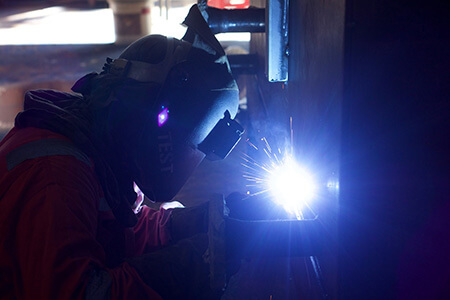Oxy-acetylene torches utilise a combustion reaction between acetylene and oxygen.

(Image: vespaburoks, Pixabay)

## Incomplete Combustion

• Incomplete combustion involves the burning of hydrocarbons where the supply of oxygen is limited.
• The products of incomplete complete combustion reactions can be carbon monoxide and water or carbon and water.
• They can be generalised by the following equations: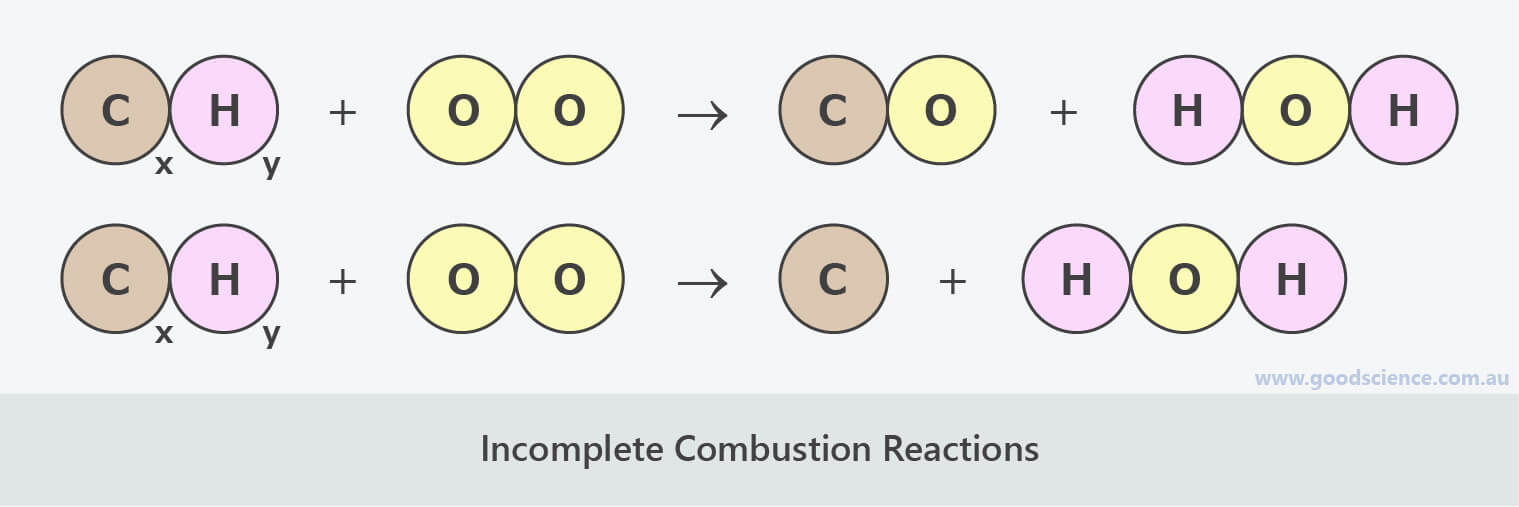• Examples of incomplete combustion reactions:
• Incomplete combustion of methane:
2 CH4 (g) + 3 O2 (g)    2 CO (g) + 4 H2O (g)
CH4 (g) + O2 (g)    C (s) + 2 H2O (g)
• Incomplete combustion of butane:
2 C4H10 (g)  +  9 O2 (g)    8 CO (g)  +  10 H2O (g)
2 C4H10 (g)  +  5 O2 (g)    8 C (s)  +  10 H2O (g)
• Incomplete combustion of ethylene:
2 C2H4 (g)  +  4 O2 (g)    4 CO (g)  +  4 H2O (g)
2 C2H4 (g)  +  2 O2 (g)    4 C (s)  +  4 H2O (g)
• In reality, most combustion reactions will produce a mixture of carbon dioxide, carbon monoxide or carbon, and water.
• The ratio of these products will depend on the supply of oxygen.Soot contains solid carbon which results from incomplete combustion of fuels.

(Image: US Environmental Protection Agency, Wikimedia Commons)

## Redox Reactions

• There are different ways that chemical reactions can be classified.
• Therefore, a particular reaction may be classified in more than one way.
• For example, combustion reactions and some combination reactions, such as corrosion reactions, can also be classified as oxidation and reduction reactions.
• An oxidation reaction can be considered as a reaction where a chemical species gains oxygen.
• A reduction reaction can be considered as a reaction where a chemical species loses oxygen.
• * When discussing redox reactions, the term ‘species’ is used rather than ‘reactant’, as it may refer to part of a substance (see below).
• Oxidation and reduction reactions always occur simultaneouslyone species is oxidised and one species is reduced.
• These reduction-oxidation reactions are commonly referred to as redox reactions.
• The species that is oxidised is known as the reducing agent, as it causes the other species to be reduced.
• The species that is reduced is known as the oxidising agent, as it causes the other species to be oxidised.
• For example, in the redox reaction below:
• Hydrogen is oxidised, as it gains oxygen to form water – it is the reducing agent.
• Simultaneously, carbon dioxide is reduced, as it loses oxygen to form carbon monoxide – it is the oxidising agent.## Redox Reactions That Do Not Involve Oxygen

• Not all redox reactions involve oxygen.
• A broader definition of a redox reaction is any reaction that involves the transfer of electrons from one chemical species to another.
• Redox reactions therefore include combustion reactions, single displacement reactions, and most combination and decomposition reactions.
• The species that is oxidised is the one which loses electrons.
• The species that is reduced is the one which gains electrons.
• For example, in the redox reaction below:
• Chloride (Cl) is oxidised, as it loses electrons to form chlorine (Cl2) – it is the reducing agent.
• Simultaneously, fluorine (F) is reduced, as it gains electrons to form fluoride (F) – it is the oxidising agent.
• Sodium ions (Na+) do not change; therefore they do not actually form part of the reaction.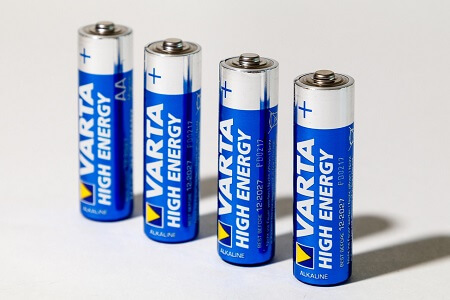Batteries generate electricity by redox reactions.

(Image: Alexei_other, Pixabay)

## Summary

• Chemical reactions can be classified in different ways, based on the way atoms and molecules are rearranged during the reaction.
• Classifying chemical reactions can make it possible to predict their products.
• Combination reactions involve two or more reactants combining to form one product.
• A  +  B    AB
• Decomposition reactions involve one reactant breaking down to form two or more products.
• AB    A  +  B
• Single displacement reactions involve an element and a compound reacting to form a different element and compound.
• A  +  BC    AC  +  B
• Double displacement reactions involve two compounds reacting to form two different compounds.
• AB  +  CD    AD  +  CB
• Complete combustion reactions involve the burning of hydrocarbons, where the supply of oxygen is not limited.
• CxHy  +  O2    CO2  +  H2O
• Incomplete combustion reactions involve the burning of hydrocarbons, where the supply of oxygen is limited.
• CxHy  +  O2    CO  +  H2O
• CxHy  +  O2    C  +  H2O
• Redox reactions involve simultaneous oxidation and reduction reactions.
• An oxidation reaction involves the loss of oxygen or the gain of electrons.
• A reduction reaction involves the gain of oxygen or the loss of electrons.
• The species that is oxidised during a redox reaction is know as the reducing agent.
• The species that is reduced during a redox reaction is know as the oxidising agent.
• Combustion reactions, single displacement reactions, and most combination and decomposition reactions are examples of redox reactions.